#Interactive Real Analysis

Next | Previous | Glossary | Map

## 7.2. Integration Techniques

### Proposition 7.2.9: The Trapezoid Rule

Let f be a twice continuously differentiable function defined on [a, b] and set
K = sup{ |f''(x) |, x[a, b] }
If h = (b - a) / n, where n is a positive integer, then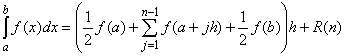where |R(n)| < K/12 (b-a) h2.
We first prove a simpler version of the trapezoid rule using the Mean Value Theorem for integrals and integration by parts.
Simple Trapezoid Rule: Let f be a function defined on the interval [0, 1] so that f is twice continuously differentiable. Then there exists a number c[0, 1] so that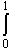f(x) dx = 1/2 (f(0) + f(1)) - 1/12 f''(c)
The trick to prove this statement is to define a function
 v(x) = 1/2 x (1 - x) which has the properties: v(x)0 for all x[0, 1] (blue) v'(x) = 1/2 - x (red) v''(x) = -1 (green)
Thenf(x) dx = -v''(x) f(x) dx
Using integration by parts with g'(x) = v''(x) we get:v''(x) f(x) dx = v'(1) f(1) - v'(0) f(0) -v'(x) f'(x) dx =
= -1/2 f(1) - 1/2 f(0) -v'(x) f'(x) dx
Again using integration by parts with g'(x) = v'(x) we get:v'(x) f'(x) dx = v(1) f'(1) - v(0) f'(0) -v(x) f''(x) dx =
-v(x) f''(x) dx =
- f''(c)v(x) dx = - f''(c) 1/12
where we used the Mean Value Theorem for Integration with some number c inside the interval [0, 1]. Taking everything together (careful with the negative signs) we then have:f(x) dx = 1/2 f(1) + 1/2 f(0) +v'(x) f'(x) dx =
= 1/2 f(1) + 1/2 f(0) - 1/12 f''(c)
which proves the simple Trapezoid Rule.

To prove the general Trapezoid Rule, assume that f is defined on [a, b]. Let h = (b - a) / n, pick an integer j, and define the function u(x) = a + jh + xh for x[0, 1]. The composite function g(x) = f(u(x)) is twice continuously differentiable and defined on the interval [0, 1] so that the simple trapezoid rule applies:g(x) dx = 1/2 g(0) + 1/2 g(1) - 1/12 g''(c)
But g(0) = f(u(0)) = f(a +jh), g(1) = f(u(1)) = f(a + (j+1)h), and g''(x) = h2 f''(x). Moreover
1/2 g(0) + 1/2 g(1) - 1/12 g''(c) =g(x) dx =f(u(x)) dx =
= 1/hf(u(x)) u'(x) dx = 1/h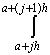f(u) du
Therefore: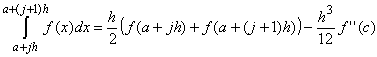Summing this equation from j = 0 to j = n-1 gives: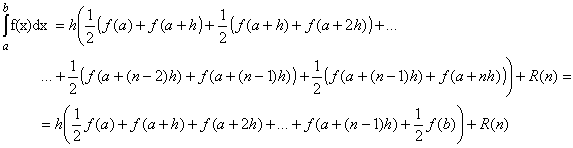where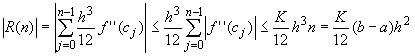which finishes the proof.
Next | Previous | Glossary | Map